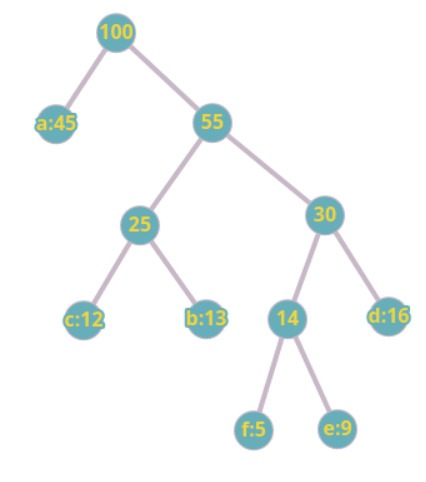Algorithms - Greedy

page 1 out of 2

Question 1

Frequency of the characters is as follows

a-45, b- 13, c- 12, d-16, e-19, f-5.Construct a tree using Huffman coding. Which of the following is correct?

Question 2

For the below tree compute the codeword for c?

Question 3

Which of the following can be done through Greedy Algorithm?

Question 4

What is the worst time complexity to find shortest path possible from One node to another node (source and destination are fixed)?

Question 5

Which of the following is correct?

Question 6

Knapsack problem can be solved using

Question 7

What is the Best approach to implement Huffman coding?

Question 8

Worst case code of the Huffman coding will be

Question 9

What is the running time complexity of Huffman code?

Question 10

In Huffman coding, data is always stored at leaves

page 1 out of 2

Oops!!

To view the solution need to Login

Score : 0 / 0
L
o
a
d
i
n
g
.
.
.